July 17, 2019

# Introduction

• Greedy algorithms
• Always makes the choice that look best at the moment
• Decision point of the algorithm
• A locally optimal choice
• In hope that this option will lead to a globally optimal solution
• They don’t always yield optimal solutions
• Although they do for many problems

# Elements of a Greedy Algorithm

• Optimal substructure
• Greedy choice property

# Greedy Choice Property

• Takes a local optimal decision at each step
• The global optimal solution doesn’t depend on the solution of its subproblems
• In principle, we can get to a global optimal solution by taking local optimal decisions (although not always)
• We need to prove this
• Different from dynamic programming
• Solves problems in a Top-Down approach

# Proving that a Greedy Algorithm is Optimal

• Need to prove that taking an optimal solution at each decision step will lead us to a global optimal solution
• We start from an optimal solution
• We show that we can modify the solution by exchanging the first step for a greedy choice and that this choice reduces the problem to a similar one but smaller
• Uses induction to show that we can make a greedy choice for each step of the algorithm
• Optimal substructure exists for the problem

# Optimal Substructure

• A problem has optimal substructure if an optimal solution contains optimal solutions in its subproblems
• As in the case of dynamic programming

# The Fractional Knapsack Problem

• A thief finds $$n$$ articles in a store
• The $$i^{th}$$ article costs $$v_i$$ dollars and weights $$w_i$$ pounds
• He wants to maximize the value of a single load
• He can carry at most $$W$$ pounds in his sack for some $$W$$
• He may take fractions of the articles without exceding $$W$$, the weight limit

# The Fractional Knapsack Problem

• Which articles and in which quantity should the thief take in order to maximize the value of the load?
• Greedy algorithm
• Take as much of the article with the highest value per pound ($$\frac{v_i}{w_i}$$) as possible
• If the article with the highest value is over, take the next article with the highest value ($$\frac{v_i}{w_i}$$)
• Continue until the sack is full

# The Fractional Knapsack Problem

• Proof of the Property of the Greedy Choice
• If we have an optimal solution to the problem of the knapsack $$O = \{o_1, o_2, \dots, o_j\}$$ with $$j$$ elements
• Suppose that there exists a greedy solution with $$G = \{g_1, g_2, \dots, g_k\}$$ with $$k$$ elements ordered according to the greedy choice

# The Fractional Knapsack Problem

• Proof of the Property of the Greedy Choice
• We want to show that there exists an optimal solution $$O^{'}$$ that includes the greedy choice $$g_1$$
• CASE 1: $$g_1$$ is not fractional
• If $$g_1$$ is in $$O$$, there is nothing to prove
• If $$g_1$$ is not in $$O$$, we arbitrarily take out $$w_{g1}$$ in articles of $$O$$ and replace it with $$g_1$$ in order to produce $$O^{'}$$
• $$O^{'}$$ is a solution and is as good as $$O$$
• CASE 2: $$g_1$$ is fractional ($$K = f^*w_{g1}$$ where $$f$$ is the fraction of $$g_1$$ chosen and $$K$$ is the weight limit)
• If $$O$$ includes $$f^*w_{g1}$$ units of $$g_1$$, there is nothing to prove
• If $$O$$ doesn’t include $$g1$$, make $$O$$ include at least $$f$$ of $$g_1$$. We take out $$f^*w_{g1}$$ weight from $$O$$ arbitrarily and replace it with $$f^*w_{g1}$$ unit of $$g_1$$ to get $$O^{'}$$
• $$O^{'}$$ is a valid solution and at least as good as $$O$$
• Proof adapted from (http://www.cs.kzoo.edu/cs215/ by Nathan Sprague)

# The Fractional Knapsack Problem

• Proof of Optimal Substructure
• We have shown that there is an optimal solution $$O^{'}$$ that contains $$g_1$$
• After choosing $$g_1$$ the weight limit is $$K^{''}=K-w_{g1}$$ and the set of articles becomes $$I^{''}=I-\{g_1\}$$
• Let $$P^{''}$$ be the knapsack problem with weight limit $$K^{''}$$ and list of articles $$I^{''}$$. We need to prove $$O^{''} = O^{'} - \{ g_1 \}$$ is an optimal solution of $$P^{''}$$
• Assume that $$O^{''}$$ is not a solution of $$P^{''}$$. Let $$Q$$ be an optimal solution with higher value than $$O^{''}$$
• Let $$R=Q \cup \{g_1\}$$. The value of $$O^{'}$$ is equal to the value of $$O^{''} + g_1$$
• The value of $$R$$ is higher than the value of of $$O^{'} = O^{''} + g_1$$
• Because $$O^{'}$$ was an optimal solution, this is a contradiction
• Proof adapted from (http://www.cs.kzoo.edu/cs215/ by Nathan Sprague)

# Activity Selection Scheduling Problem

• Given a set of $$n$$ activities to be performed
• $$S = \{1, 2, \dots, n\}$$
• All activities require the same resource (i.e. the same classroom) and they use it one at a time
• Each activity $$i$$ has an initial time $$s_1$$ and a finishing time $$f_i$$, $$s_i \leq f_i$$, $$[s_i, f_i)$$
• Two activities $$i$$ and $$j$$ are compatible if they do not overlap: $$s_i \geq f_j$$ or $$s_j \geq f_i$$
• Problem Definition: Choose the largest set of compatible activities
• Assume that the input is ordered by $$f_1 \leq f_2 \leq \dots \leq f_n$$, ($$O(n \lg n))$$.

# Activity Selection Scheduling Problem

• In this example, subset $$\{a_3, a_9, a_{11}\}$$ consists of mutually compatible activities
• It is not a maximum subset, subset $$\{a_1, a_4, a_8, a_{11}\}$$ is larger
• $$\{a_1, a_4, a_8, a_{11}\}$$ is the largest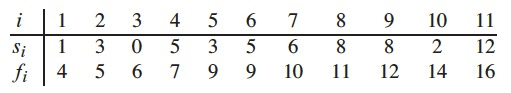# Activity Selection Scheduling Problem

• Optimal Substructure of the Activity-Selection Problem
• $$S_{ij} \rightarrow$$ set of activities
• Start after activity $$a_i$$ finishes
• Finish before activity $$a_j$$ starts
• We wish to find a maximum set of mutually compatible activities in $$S_{ij}$$
• Suppose this set is $$A_{ij}$$, it includes activity $$a_k$$
• We are left with 2 subproblems
1. Find mutually compatible activities in set $$S_{ik}$$
• $$A_{ik}= A_{ij} \cap S_{ik}$$
2. Find mutually compatible activities in set $$S_{kj}$$
• $$A_{kj}= A_{ij} \cap S_{kj}$$
• $$A_{ij}=A_{ik} \cup \{a_k\} \cup A_{kj}$$ containing $$|A_{ij}|=|A_{ik}|+|A_{kj}|+1$$ activities (mutually compatible)
• Cut-and-paste argument shows that optimal solution $$A_{ij}$$ must also include optimal solutions to the two subproblems $$S_{ik}$$ and $$S_{kj}$$
• If we could find $$A^{'}_{kj}$$ of mutually compatible activities in $$S_{kj}$$
• With $$|A^{'}_{kj}| > |A_{kj}|$$
• We could use $$A^{'}_{kj}$$ instead of $$A_{kj}$$ in a solution to $$S_{ij}$$
• We would have a set $$|A_{ik}|+|A^{'}_{kj}|+1 > |A_{ik}|+|A_{kj}|+1 = |A_{ij}|$$
• This contradicts the assumption that $$A_{ij}$$ is an optimal solution
• A symmetric argument applies to the activities in $$S_{ik}$$.
• We characterized optimal substructure
• We could solve the problem with dynamic programming
• Denote size of an optimal solution for set $$S_{ij}$$ by $$c[i,j]$$ we have the recurrence $$c[i,j]=c[i,k]+c[k,j]+1$$
• Need to examine all activities in $$S_{ij}$$ to find activity $$a_k$$
• We could memoize the recursive algorithm
• We would be missing an important characteristic of the activity-selection problem
• The greedy choice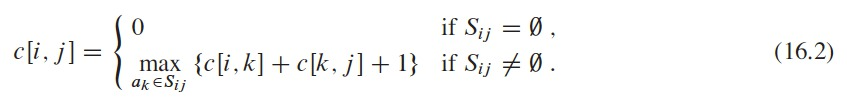# Activity Selection Scheduling Problem

• Making the greedy choice
• It would be good if we could choose an activity to add to the optimal solution without having to solve all subproblems
• Saving us from considering all choices in the recurrence (16.2)
• We only need to consider one choice, that is, the greedy choice
• Intuitively
• Choose activity that leaves the resource available (as most as possible)
• Choose activity with the earliest finish time
• Activities are sorted in monotonically increasing order by finish time
• Then, greedy choice is $$a_i$$
• There are other ways to make a greedy choice for this problem
• After choosing $$a_1$$ the problem is: finding activities starting after $$a_1$$ finishes (compatible activities)
• Activity selection problem exhibits optimal substructure
• Let $$S_k = \{a_i \in S : s_i \geq f_k\}$$ be the activities that start after activity $$a_k$$ finishes
• If we make greedy choice $$a_1$$
• $$S_1$$ is the only problem to solve next
• Is this greedy choice correct?

# Activity Selection Scheduling Problem

• Theorem 16.1
• Consider any nonempty subproblem $$S_k$$, and let $$a_m$$ be an activity in $$S_k$$ with the earliest finish time. Then $$a_m$$ is included in some maximum-size subset of mutually compatible activities of $$S_k$$.
• Proof
• Let $$A_k$$ be a maximum-size subset of mutually compatible activities in $$S_k$$
• Let $$a_j \in A_k$$, be the activity with the earliest finish time
• If $$a_j = a_m$$, nothing to do because $$a_m$$ is in some maximum-size subset of mutually compatible activities of $$S_k$$
• If $$a_j \neq a_m$$
• Let set $$A^{'} = A_k - \{a_j\} \cup \{a_m\}$$ be $$A_k$$ substituting $$a_j$$ for $$a_m$$
• Activities in $$A_k$$ are disjoint
• $$a_j$$ is the first activity in $$A_k$$ to finish
• $$f_m \leq f_j$$
• Since $$|A^{'}_k| = |A_k|$$
• We conclude that $$A^{'}_k$$ is a maximum-size subset of mutually compatible activities of $$S_k$$ that includes $$a_m$$

# Activity Selection Scheduling Problem

• We don’t need to solve the activity-selection problem with dynamic programming
• We can repeatedly choose the activity that finishes first
• Keep only compatible activities
• Repeat until no activities remain
• The algorithm doesn’t have to work bottom-up
• It can work top-down
• Make a choice and then solve the subproblem
• Instead of bottom-up, solving subproblems before making a choice

# Activity Selection Scheduling Problem

• Recursive greedy algorithm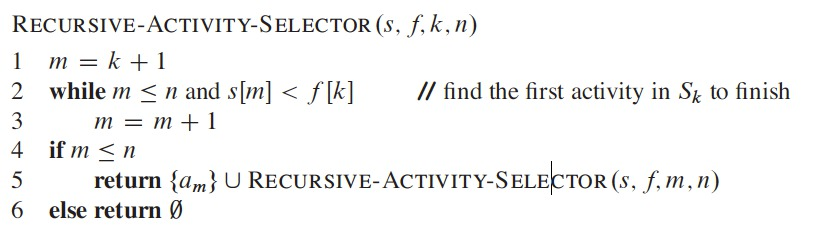# Activity Selection Scheduling Problem

• Recursive greedy algorithm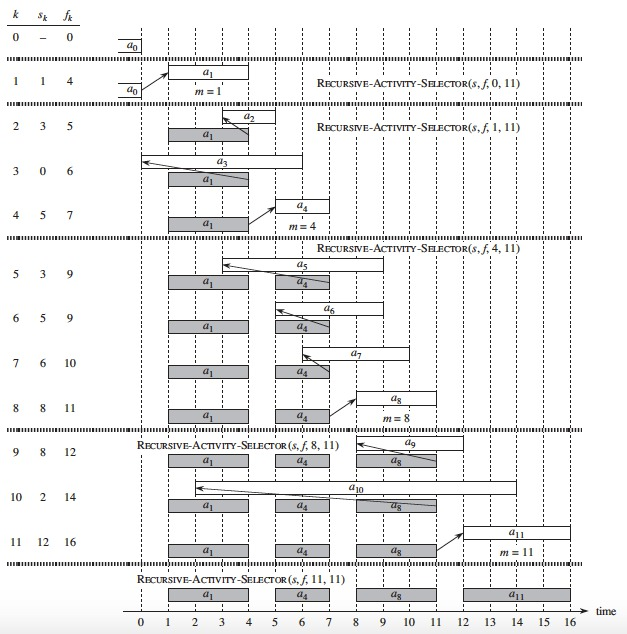# Activity Selection Scheduling Problem

• Recursive greedy algorithm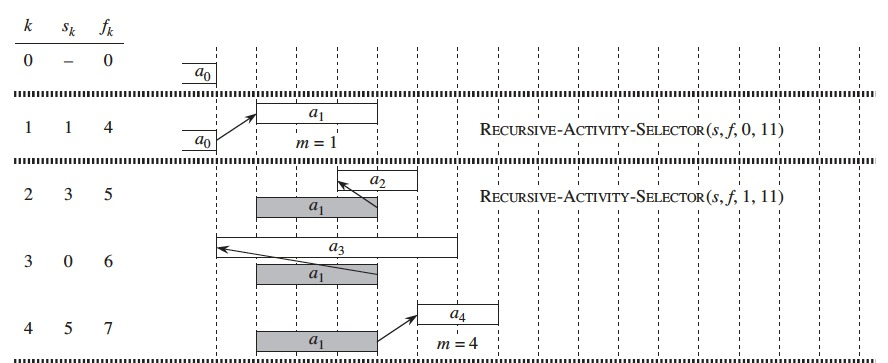# Activity Selection Scheduling Problem

• Recursive greedy algorithm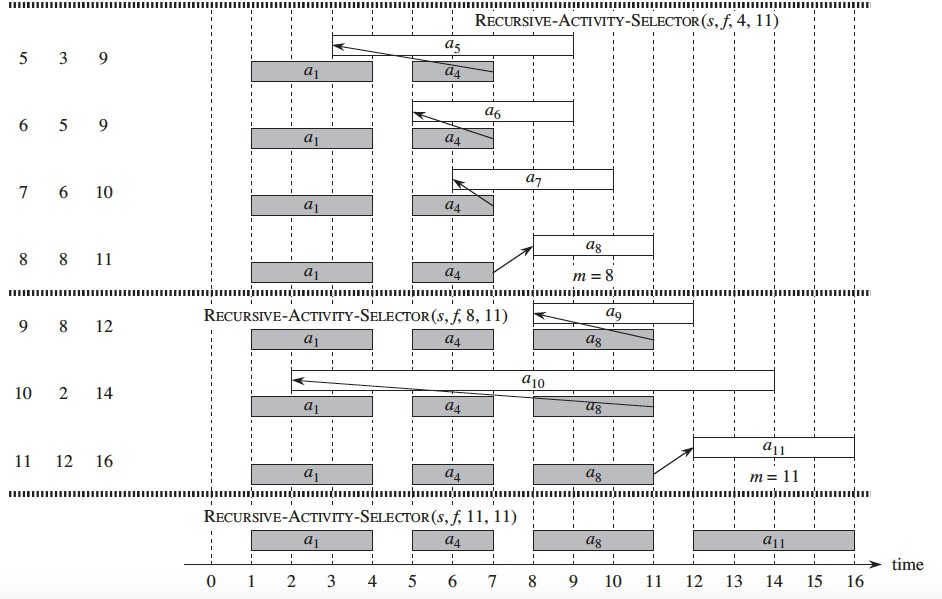# Activity Selection Scheduling Problem

• Recursive greedy algorithm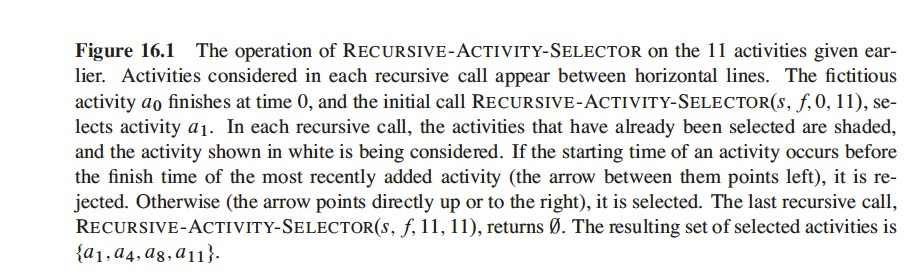# Activity Selection Scheduling Problem

• Iterative greedy algorithm
• Also assume input activities ordered by monotonically increasing finish time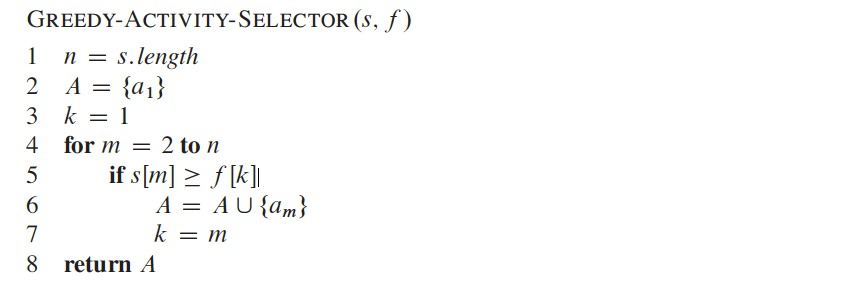# Elements of the Greedy Strategy

• Steps we followed for generating a greedy solution for the Activity-Selection problem:
1. Determine the optimal substructure of the problem
2. Develop a recursive solution
3. Show that if we make the greedy choice, then only one subproblem remains
4. Prove that it is always safe to make the greedy choice
5. Develop a recursive algorithm that implements the greedy strategy
6. Convert the recursive algorithm to an iterative algorithm
• We kept in mind the dynamic programming technique while solving the problem
• We could have designed our optimal substructure with the greedy choice in mind
• Our choice leave only one subproblem to solve
• Define subproblems of the form $$S_k$$
• Prove that a greedy choice leads to an optimal solution
• Geedy choice: first activity $$a_m$$ to finish in $$S_k$$ + optimal solution to remaining $$S_m$$

# Elements of the Greedy Strategy

• The greedy technique design steps
1. Approach the optimization problem with the greedy choice, and left with one subproblem to solve
2. Prove there is always an optimal solution to the original problem by making the greedy choice
• The greedy choice is always safe
3. Demonstrate optimal substructrue
• After making the greedy choice, we are left with a subproblem that if we combine an optimal solution to the subproblem with the greedy choice just made, we optimally solve the original problem
• 2 Key ingredients
• Greedy-choice property
• Optimal substructure
• Demonstrate the problem has these 2 properties

# Greedy-choice Property

• Assemble an optimal solution to a problem
• Making locally optimal (or greedy) choices
• At each step, we make the choice that looks best in the current problem
• We don’t consider results from different subproblems

# Greedy-choice Property

• Difference with Dynamic Programming (DP)
• In DP we make choice at each step but this choice depends on solution to subproblems
• We solve them in a bottom-up way, from smaller to larger subproblems
• Or top-donw by memoizing, we still solve smaller problems first
• Greedy algorithms usually proceed in top-down, reducing the problem size at each step
• We need to prove that the greedy choice leads to a globally optimal solution
• We can make the greedy choice more efficient
• i.e. in activity selection problem
• Sorting activities in monotonically increasing order of finish times
• We examine each activity only once
• We can use a data structure (i.e. a priority queue) to make the greedy choices quickly

# Greedy-choice Property

• The greedy-choice doesn’t always work
• It doesn’t work for the 0-1 knapsack problem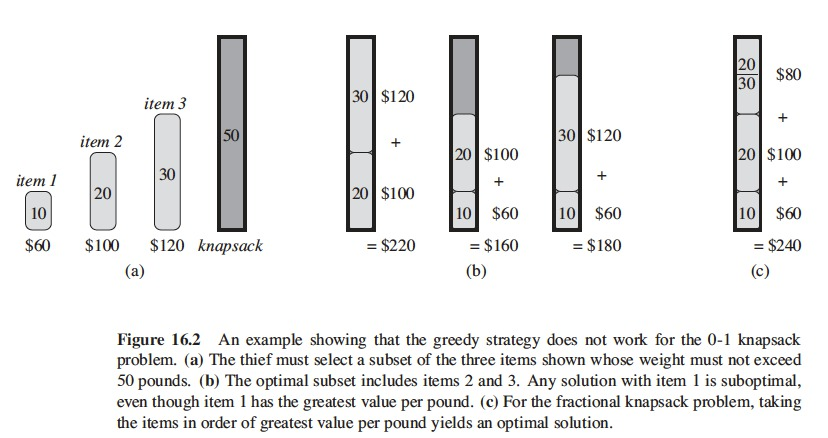# Huffman Codes

• Huffman codes are used for data compression
• 20% to 90% savings are typical
• Greedy algorithm
• Uses the characters frequency to create the codes
• Find optimal codes of variable length for characters
• Given a file with 100,000 characters, with 6 different characters: a, b, c, d, e, f
• We want to design a binary character code, or code for short
• Each character represented by a unique binary string, a codeword
• If we use a fixed-length code, we require 3 bits to represent 6 characters
• a=000, b=001, $$\dots$$, f=101
• Requiring 300,000 bits to code the whole file
• A variable-length code can do better than the fixed-length code
• Give short codewords to frequent characters
• Give long codewords to infrequent characters
• Code requires:
• $$(45 \times 1 + 13 \times 3 + 12 \times 3 + 16 \times 3 + 9 \times 4 + 5 \times 4) \times 1,000 = 224,000$$ bits
• Savings of approx. 25%
• This is an optimal character code for this file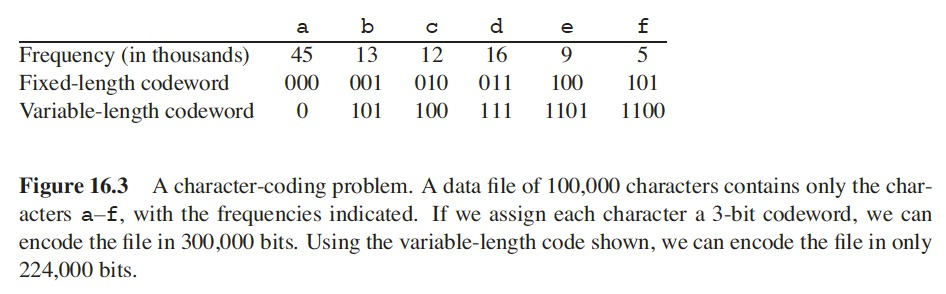# Huffman Codes

• Prefix codes: no codeword is a prefix of some other codeword
• A prefix code can achieve optimal data compression among any character code
• Encoding is simple
• Concatenate the codewords representing each character
• The 3-character file $$abc \rightarrow 0 \cdot 101 \cdot 100 = 0101100$$
• $$\cdot$$ denotes concatenation
• Prefix codes simplify decoding
• Codeword that begins the encoded file is unambiguous
• We decode the initial word, and repeat the process with the remainder of the encoded file

# Huffman Codes

• $$001011101$$ parses as $$0 \cdot 0 \cdot 101 \cdot 1101$$
• Decodes to $$aabe$$
• Decoding process requires a convenient representation
• Find easy way to pick off the initial codeword
• Binary tree, leaves are the characters
• Not binary search trees, i.e. leaves aren’t sorted
• A codeword of a character is the path from the root to the character
• $$0$$, the left child
• $$1$$, the right child